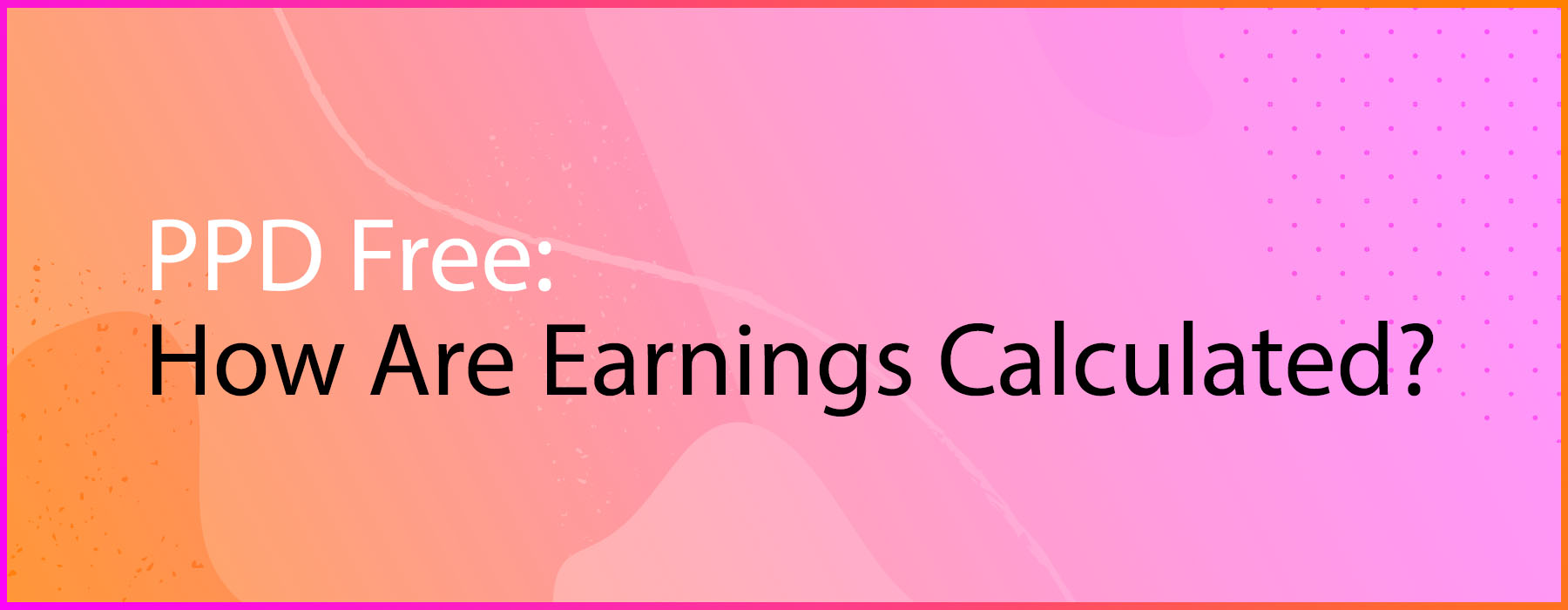#### Would you like to use Vecteezy in English?

View in different language

# How are PPD Free Earnings Calculated?# PPD Free Earnings Overview

Unlike PPD Pro earnings, PPD (Pay Per Download) Free earnings are calculated based on the total number of downloads a contributor receives across their portfolio.

This model is calculated based on a set rate: \$5.00 paid per 1,000 free downloads.

# Calculating PPD Free Earnings

To calculate PPD Free Earnings, Vecteezy takes the total number of downloads a contributor receives and multiplies this amount by \$0.005.

• Total downloads x \$0.005 = amount earned

We use this formula for each contributor monthly to calculate total PPD Free contributor earnings.

# PPD Free Earnings Examples

Here are two examples that illustrate PPD Free payouts:

Contributor A:

Receives 10,000 downloads in a month and earns \$50.00 for that month

• 10,000 downloads received x \$0.005 = \$50.00

Contributor B:

Receives 250,000 downloads in a month and earns \$1,250.00 for that month

• 250,000 total downloads x \$0.005 = \$1,250.00Learn more about our PPD Free and PPD Pro Contributor programs here.

Interested in becoming a contributor for Vecteezy? Apply Here!